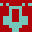# Euler Problem 303

http://projecteuler.net/problem=303

For a positive integer n, define f(n) as the least positive multiple of n that, written in base 10, uses only digits <= 2.

Thus f(2)=2, f(3)=12, f(7)=21, f(42)=210, f(89)=1121222.

find sum(1..10000,f(n)/n)

Solution: generate all f(n) of the desired form ("cool numbers"), starting with the smallest, and see if any of the remaining n divide it.

This program conforms to Forth-94

## by AntonErtl